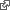History of Boolean

Dijkstra acknowledges Booleans as one of three significant contributions introduced with Algol 60 which he considers the founding of computing science. transcriptquote

The next milestone of ALGOL 60 that I must mention is ALGOL 60’s introduction of “boolean” as a full-blown type, i.e. not only with boolean expressions but also with boolean constants, variables, arrays, procedures and parameters. From a programming point of view it was not so shocking: besides “integers” whose values can be represented by some finite number of bits, one can introduce a type for which a single bit suffices, but in a wider culture it is making a major difference.

Even for programmers, who saw that there was no implementation problem at all, the introduction of the boolean variable was --and probably still is-- a big leap, as is convincingly illustrated by the fact that for years one would still find even in published programs clauses like:

if c = true then ....

when of course

if c then .....

would suffice.

Evolutions do take time. Mathematicians have used boolean expressions at least since 1557, when Robert Recorde introduced the equality symbol “=” as infix operator, but a formula like “n=5” was not recognized as an expression, not syntactically and not semantically, because for lack of the type boolean, “n=5” was not something that could have a value.

This changed in 1854 when George Boole introduced the type we now name after him, an invention, however, that the mathematical community had not yet absorbed a century later: for the average mathematician, and even for the not so average one, in 1954, a boolean expression was not something with a value, but as in 0 <= n <= 10, a condition or a statement of fact, and, say, 2+3=7 was not a complicated way of writing the constant false, it was just wrong, an attitude that precludes the manipulation of uninterpreted formulae containing boolean subexpressions. I now see more and more people doing calculational mathematics such as formulating a theorem as a boolean expression and calculating that its value is true.

This new style of doing mathematics embodies a major simplification of the mathematical argument. Computing Science developed it because it needed and had the potential to do so; ALGOL 60’s promotion of the type boolean has played a historically significant role in that development.

.